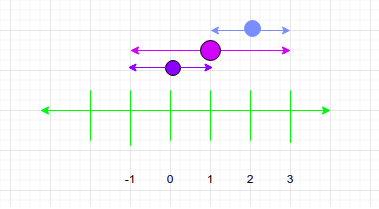Problem title
Difficulty
Avg time to solve

Container With Most Water
Moderate
15 mins
Bubble Sort
Easy
10 mins
Maximum Product Subarray
Moderate
25 mins
Guess Price
Easy
10 mins
Partition Equal Subset Sum
Moderate
25 mins
Minimum Fountains
Easy
10 mins
Family Structure
Easy
10 mins
House Robber II
Moderate
15 mins
Merge K Sorted Arrays
Moderate
15 mins
Sum root to leaf
Easy
10 mins34

# Minimum Fountains

Difficulty: EASY
Avg. time to solve
10 min
Success Rate
80%

Problem Statement

#### Note:

``````1. 0-based indexing is used in the array.
2. We only care about the garden from 0 to 'N' only. So if i - 'ARR'['i'] < 0 or i + 'ARR'['i'] > 'N', you may ignore the exceeding area.
3. If some fountain covers the garden from position 'A' to position 'B', it means that the water from this fountain will spread to the whole line segment with endpoints 'A' and 'B'.
``````
##### Input Format:
``````The first line of the input contains an integer 'T', denoting the number of test cases.

The first line of each test case contains the integer 'N', denoting the size of the garden.

The second line of each test case contains 'N' + 1 space-separated integers denoting the array elements.
``````
##### Output Format:
``````For each test case, print a single integer that corresponds to the minimum number of fountains to be activated.
``````

#### Note :

``````You do not need to print anything, it has already been taken care of. Just implement the given function.
``````
##### Constraints:
``````1 <= 'T' <= 50
1 <= 'N' <= 10^4
1 <= 'ARR'[i] <= 'N'

Where 'ARR[i]' represents the elements at 'i'th index.

Time Limit: 1 sec
``````
##### Sample Input 1:
``````2
2
1 2 1
4
2 1 2 1 1
``````
##### Sample Output 1:
``````1
1
``````
##### Explanation for Sample Input 1:``````For the first test case, as shown in the figure,
Fountain at i = 0 can cover area from (-1,1).
Fountain at i = 1 can cover area from (-1, 3).
Fountain at i = 2 can cover the area from (1, 3).
So if we activate the fountain on index = 1, we will be able to cover the whole garden.

For the second test case,  we can just activate the fountain on index 2 as it will cover all the positions from 0 to 4
``````
##### Sample Input 2:
``````1
4
2 1 1 2 1
``````
##### Sample Output 2:
``````2
``````
##### Explanation for Sample Input 2:
``````We can activate the fountains on index 0 and 3 to cover the whole garden.
``````Console